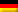## Module RGAS

#### Fundamentals:

In all thermal calculations, e.g. in steam generator design, the properties of the resulting exhaust gases must be known.

The properties of gas mixtures depend on pressure, temperature and composition.

#### Program features

The RGAS module uses statistical combustion calculation methods to calculate the composition of a flue gas as a function of the lower calorific value Hu and the measured CO2 content for solid fuels, fuel oils or natural gases.

With this composition (CO2, H2O, N2, O2) the thermal conductivity, the dynamic viscosity, the specific heat capacity (true and integral) as well as the Prandtl number are calculated after input of pressure and temperature with the approximate polynomials mentioned in the literature.

Other properties such as molar mass, enthalpy, specific gas constant, density etc. are also calculated.

If the composition of the flue gas is known (in volume % wet), the properties can also be calculated directly.

The following physical properties are determined:

• Density
• Standard density
• True and mean specific heat capacity
• Dynamic and kinematic viscosity
• Thermal conductivity
• Prandtl number
• Thermal diffusivity
• Specific enthalpy
• Specific volume
• Molecular weight
• Specific gas constant

#### Price: see price list

Dieser Beitrag ist auch verfügbar auf:Deutsch (German)